# GMAT Math : Arithmetic

## Example Questions

← Previous 1 3 4 5 6 7 8 9 31 32

### Example Question #1 : Properties Of Integers

Is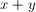odd?

(1)is odd

(2)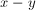is even

Statement (2) ALONE is sufficient, but statement (1) alone is not sufficient.

Statements (1) and (2) TOGETHER are NOT sufficient.

EACH statement ALONE is sufficient.

Statement (1) ALONE is sufficient, but statement (2) alone is not sufficient.

BOTH statements TOGETHER are sufficient, but NEITHER statement ALONE is sufficient.

Statement (2) ALONE is sufficient, but statement (1) alone is not sufficient.

Explanation:

For statement (1), we only know thatis odd but we have no idea about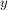. Ifis odd, thenis even. Ifis even, thenis odd. Therefore we have no clear answer to the question using this condition. For statement (2), sinceis even, we know thatandare either both odd or both even, therefore we know for sure thatis even and the answer to this question is “no”.

### Example Question #2 : Properties Of Integers

Ifis an integer and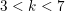, what is the value of?

(1)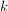is a factor of 20.

(2)is a factor of 24.

Statement (1) ALONE is sufficient, but statement (2) alone is not sufficient.

EACH statement ALONE is sufficient.

Statements (1) and (2) TOGETHER are NOT sufficient.

Statement (2) ALONE is sufficient, but statement (1) alone is not sufficient.

BOTH statements TOGETHER are sufficient, but NEITHER statement ALONE is sufficient.

BOTH statements TOGETHER are sufficient, but NEITHER statement ALONE is sufficient.

Explanation:

From statement (1), we know that the possible value ofwould be 4 and 5. From statement (2), we know that the possible value ofwould be 4 and 6. Putting the two statements together, we know that only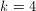satisfies both conditions. Therefore both statements together are sufficient.

### Example Question #3 : Properties Of Integers

Ifis a positive integer, isdivisible by 6?

1. The sum of the digits ofis divisible by 6

2.is even

BOTH statements TOGETHER are sufficient, but NEITHER statement ALONE is sufficient.

EACH statement ALONE is sufficient.

Statement (1) and (2) TOGETHER are NOT sufficient to answer the question asked, and additional data are needed.

Statement (1) ALONE is sufficient, but statement (2) alone is not sufficient.

Statement (2) ALONE is sufficient, but statement (1) alone is not sufficient.

BOTH statements TOGETHER are sufficient, but NEITHER statement ALONE is sufficient.

Explanation:

Statement 1: Numbers whose digits sum to a number divisible by 3 are divisible by 3, but the same does not apply to sums of 6. This indicates thatis divisble by 3 but is not sufficient at provingis divisible by 6.

Statement 2: Though all multiples of 6 are even, not all even numbers are multiples of 6.

Together: The fact thatis a multiple of 3 and even is sufficient evidence for the conclusion that x is divisible by 6.

### Example Question #4 : Properties Of Integers

Ispositive, negative, or zero?

1)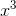is positive.

2)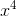is positive.

Statement 2 ALONE is sufficient, but Statement 1 alone is not sufficient.

Statements 1 and 2 TOGETHER are not sufficient.

Statement 1 ALONE is sufficient, but Statement 2 alone is not sufficient.

BOTH statements TOGETHER are sufficient, but neither statement ALONE is sufficient.

EACH statement ALONE is sufficient.

Statement 1 ALONE is sufficient, but Statement 2 alone is not sufficient.

Explanation:raised to an odd power must have the same sign as, so, ifis positive, thenis also positive. But either a positive number or a negative number raised to an even power must be positive. Therefore,being positive is inconclusive.

Therefore, the correct choice is that Statement 1, but not Statement 2, is sufficient.

### Example Question #5 : Properties Of Integers

The greatest common factor of 32 and a numberis 16. What is?

1) 3 is also a factor of.

2) 5 is also a factor of.

EITHER Statement 1 or Statement 2 ALONE is sufficient to answer the question.

BOTH statements TOGETHER are sufficient to answer the question, but NEITHER statement ALONE is sufficient to answer the question.

BOTH statements TOGETHER are NOT sufficient to answer the question.

Statement 2 ALONE is sufficient to answer the question, but Statement 1 ALONE is not sufficient.

Statement 1 ALONE is sufficient to answer the question, but Statement 2 ALONE is not sufficient.

BOTH statements TOGETHER are NOT sufficient to answer the question.

Explanation:

Thatcannot be determined, even if both statements are known to be true, can be proved by demonstrating two examples ofthat fit these conditions. We can do this by comparing the prime factorizations of 32 and.

Example: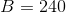To find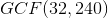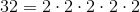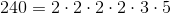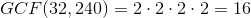Example: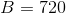To find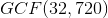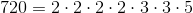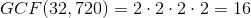In each case, 3 and 5 are factors of, and in each case,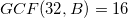The answer is that both statements together are insufficient.

### Example Question #6 : Properties Of Integers

What is the area of a rhombus in square inches?

1) One of its angles measures2) One of its sides measures 10 inches

Statement 2 ALONE is sufficient to answer the question, but Statement 1 ALONE is not sufficient.

Statement 1 ALONE is sufficient to answer the question, but Statement 2 ALONE is not sufficient.

EITHER Statement 1 or Statement 2 ALONE is sufficient to answer the question.

BOTH statements TOGETHER are sufficient to answer the question, but NEITHER statement ALONE is sufficient to answer the question.

BOTH statements TOGETHER are NOT sufficient to answer the question.

BOTH statements TOGETHER are sufficient to answer the question, but NEITHER statement ALONE is sufficient to answer the question.

Explanation:

As is true of any other parallelogram, the area of the rhombus is the base multiplied by the height. The common sidelength alone can be used to determine the base, but without the angles, the height cannot be determined. Using trigonometry, the angle can be used to determine the height relative to the base, but without the base, the height is unknown.

If we know both of the given statements, then part of one base, an altitude from an endpoint of the opposite base, and one adjacent side form a 30-60-90 triangle. The hypotenuse of that triangle is 10 inches, and the altitude is half that, or 5 inches. This makes the area 50 square inches.

The answer is that both statements together are sufficient to answer the question, but neither statement alone.

### Example Question #1 : Properties Of Integers

Data Sufficiency Question

Out of 100 students, 60 took French and 25 took German. How many students took neither?

1. 15 students took Spanish

2. 7 students took both French and German

statements 1 and 2 together are not sufficient, and additional data is needed to answer the question

statement 2 alone is sufficient, but statement 1 alone is not sufficient to answer the question

statement 1 alone is sufficient, but statement 2 alone is not sufficient to answer the question

each statement alone is sufficient

both statements taken together are sufficient to answer the question, but neither statement alone is sufficient

statement 2 alone is sufficient, but statement 1 alone is not sufficient to answer the question

Explanation:

Statement 1 does not tell us anything about the number of students taking French or German. The information from statement 2 is sufficient, if 60 took French, 25 took German, and 7 took both, we can calculate the number that took neither.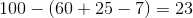### Example Question #8 : Properties Of Integers

Ifandare both integers, evaluate.

Statement 1: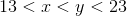.

Statement 2:andare both prime integers.

Statement 2 ALONE is sufficient to answer the question, but Statement 1 ALONE is NOT sufficient to answer the question.

BOTH statements TOGETHER are insufficient to answer the question.

EITHER statement ALONE is sufficient to answer the question.

Statement 1 ALONE is sufficient to answer the question, but Statement 2 ALONE is NOT sufficient to answer the question.

BOTH statements TOGETHER are sufficient to answer the question, but NEITHER statement ALONE is sufficient to answer the question.

BOTH statements TOGETHER are sufficient to answer the question, but NEITHER statement ALONE is sufficient to answer the question.

Explanation:

There are infinitely many primes, and several integers between 13 and 23, so knowing just one of these statements is not enough. But only two integers in the stated range - 17 and 19 - are prime, so knowing both statements tells you thatandare 17 and 19, respectively. Subsequently, you can add them to get 36.

### Example Question #9 : Properties Of Integers

If a positive integeris divided by 2, what is the remainder?

Statement 1: Ifis divided by 2, the remainder is 1.

Statement 2: Ifis divided by 4, the remainder is 3.

BOTH statements TOGETHER are insufficient to answer the question.

Statement 2 ALONE is sufficient to answer the question, but Statement 1 ALONE is NOT sufficient to answer the question.

BOTH statements TOGETHER are sufficient to answer the question, but NEITHER statement ALONE is sufficient to answer the question.

EITHER statement ALONE is sufficient to answer the question.

Statement 1 ALONE is sufficient to answer the question, but Statement 2 ALONE is NOT sufficient to answer the question.

EITHER statement ALONE is sufficient to answer the question.

Explanation:

The question is the same as asking whetheris odd or even.

Statement 1 says that the square ofis odd. If we know this, then we know thatis odd, since the square of an even number is even.

Statement 2 says thatis 3 greater than a multiple of 4; this makesodd.

Therefore, either statement alone tells us thatis an odd number.

### Example Question #10 : Properties Of Integers

What is the last digit of a positive integer?

Statement 1: The last digit of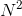is 1.

Statement 2: The last digit of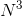is 1.

Statement 1 ALONE is sufficient to answer the question, but Statement 2 ALONE is NOT sufficient to answer the question.

Statement 2 ALONE is sufficient to answer the question, but Statement 1 ALONE is NOT sufficient to answer the question.

BOTH statements TOGETHER are sufficient to answer the question, but NEITHER statement ALONE is sufficient to answer the question.

BOTH statements TOGETHER are insufficient to answer the question.

EITHER statement ALONE is sufficient to answer the question.

Statement 2 ALONE is sufficient to answer the question, but Statement 1 ALONE is NOT sufficient to answer the question.

Explanation:

If the last digit ofis 1, then the last digit ofis either 1 or 9.

If the last digit ofis 1, however, the last digit ofmust be 1.

← Previous 1 3 4 5 6 7 8 9 31 32

Tired of practice problems?

Try live online GMAT prep today.